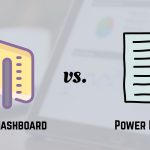[email protected] +1 203-349-9909 / +91-8080042523

July 14, 2017

Calculate Elapsed Time in Excel

We want to calculate elapsed time in excel i.e. Add or Subtract hours, minutes and seconds to the time. How to calculate elapsed time in Excel? Use the following formula TIME (HOURS, MINUTES, SECONDS) to calculate elapsed time in excel. After entering the formula, you may require changing the cell format as per your requirement. In...

Excel VLOOKUP Function

Before we learn how to use Excel VLOOKUP function, we must learn when do we use Excel VLOOKUP function? Task: Imagine if you have to find when does train no. 6948 departs in the schedule chart above? Your approach: You will scan the first column top to bottom. When you find the same train no.,...

Loop Multiple Sheets and Merge Data using Excel VBA Worksheets

In this blog article, we will be covering how to loop through multiple sheets and merge data in Excel VBA Worksheets as per our requirement. Just imagine you have a lot of files in a folder and for each file, you have 3 sheets. Let’s Start how to merge data in VBA Worksheets. Now If I...